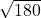## Can someone tell me what Pythagorean theorem is? I really need help.

Question

Can someone tell me what Pythagorean theorem is? I really need help.

in progress 0
7 months 2021-07-25T04:51:10+00:00 1 Answers 3 views 0

The sum of the squares of the two sides equals the square of the hypotenuse (a² + b² = c²).

Let’s say you have a triangle with a 90º angle (also known as a right angle). The two equal legs are a and b while the hypotenuse is the longer side. Hypotenuse is and always will be c.

Here’s a problem:

c ⊿ a (12)

b (6)

C is missing, we have to find C, so here’s what we do:

Step 1: You square all the numbers in the equation

12² + 6² = c²

144 + 36 = c

Step 2: You add 144 + 36 and you get 180

Step 3: Then you find the square root >>and you get ≈13.4

Now sometimes, the a or b would be missing, for example:

c (13)⊿ a

b (5)

A is missing (when a or b is missing, use the following formula, do not use the same formula when c is missing)

Step 1: You square all the numbers in the equation

a² + 5² = 13²

a + 25 = 169

For this next part, you must subtract a or b (in this case, b) on both sides, for example:

25 – 25 = 169 – 25

25 – 25 cancels itself out so now we subtract 169 by 25 and we get 144.

Then we find the square root of 144 and we get 12

I hope this helps 🙂